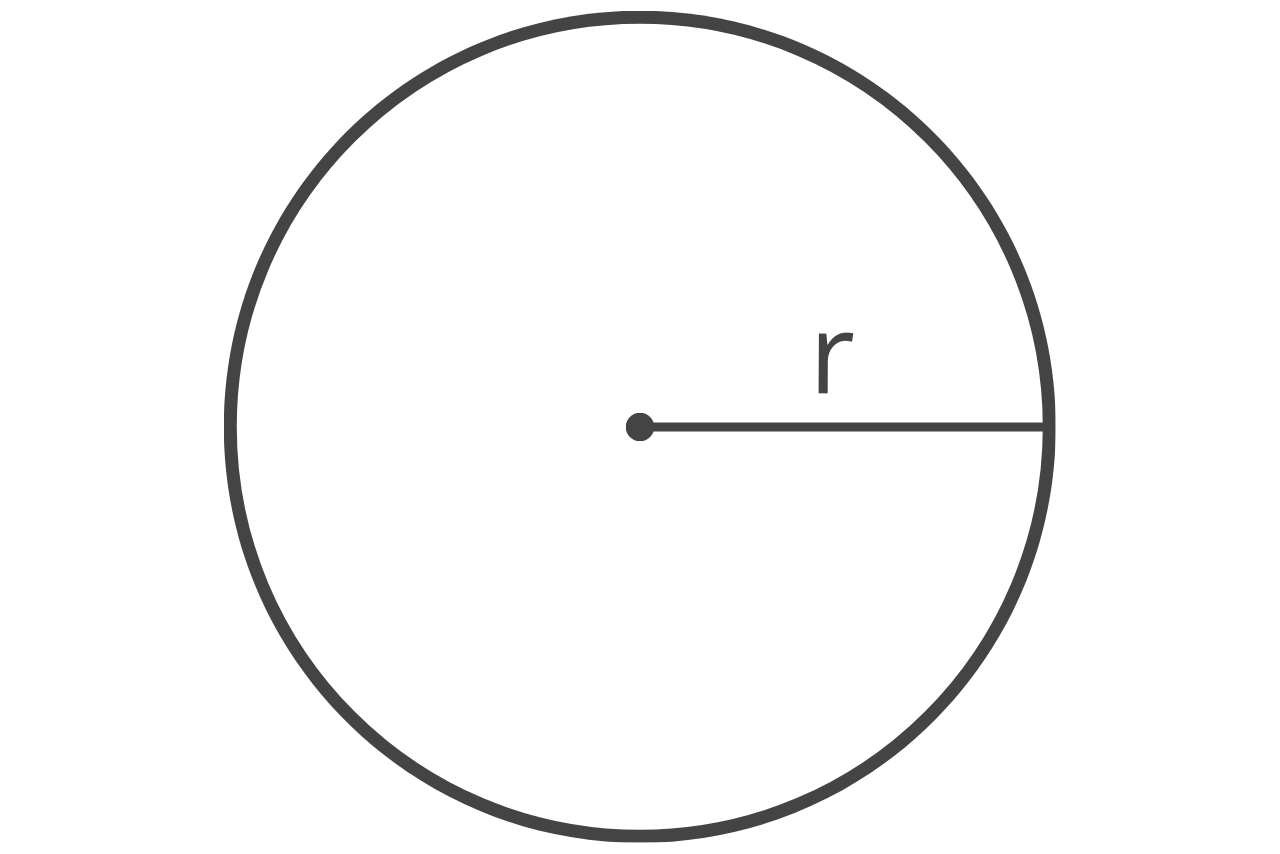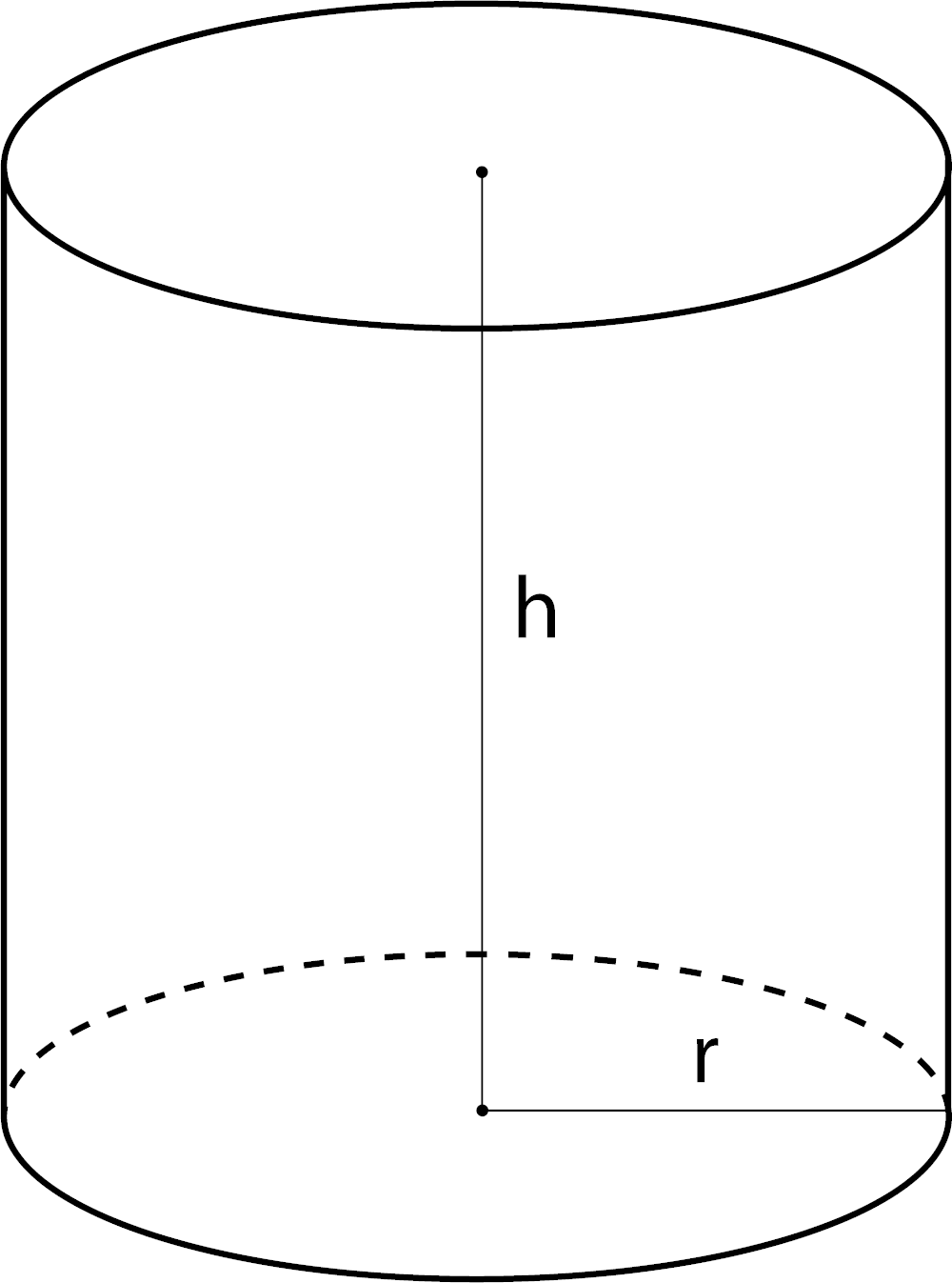# Cylinder Cubic Yardage Calculator

Calculate the volume in cubic yards for a cylinder given its height and diameter.

Optionally enter the price per cubic yard
\$

## Material Estimate:

Cubic Yards
Estimated Material Cost

Cost
Learn how we calculated this below

## How to Find the Cubic Yardage of a Cylinder

This calculator is perfect for finding the volume of a cylinder in cubic yards, given the height of the cylinder and the radius or diameter of its base. This is useful for determining cubic yardage needed for concrete projects, filling sonotubes, posts, or footings, building and construction projects, or landscaping projects.

This is also helpful for anyone trying to find the volume of a cylinder. Estimate the cost for materials to fill the cylinder by entering a price per cubic yard.

The volume of a cylinder is found by multiplying the radius squared times π times the height. The formula looks like this:

volume = π × r2 × h

### Steps to Find the Volume of a Cylinder in Cubic Yards

To calculate the volume of a cylinder in cubic yards using this formula, follow these steps.

• Find the radius and height. If the diameter is known, then divide the diameter by 2 to get the radius, or use a radius calculator. The radius and height should be in yards; if you have other measurements convert to yards
• Multiply the radius of the cylinder by itself, e.g. r2
• Multiply times π to get the area of the cylinder’s base
• Multiply times the height of the cylinder to get the volume
• If you are trying to calculate the total cost of materials needed to fill the cylinder, then multiply the cubic yardage by the price per cubic yard to get the cost of materials
• Use a tool such as our cylinder cubic footage calculator if you need results in cubic feet

It’s essential to convert each measurement to yards, or the resulting volume will not be in cubic yards. For example, if the measurements are in inches, then the result will be in cubic inches, and if they are in feet, then the result will be in cubic feet.

### Circle Area Formula

area = π × (diameter ÷ 2)2
area = π × r2

π = 3.14159265359### Cylinder Volume Formula

volume = π × (diameter ÷ 2)2 × height
volume = π × r2 × h

π = 3.14159265359